TitleCollege Algebra
Tutorial 37:
Synthetic Division and
the Remainder and Factor TheoremsLearning Objectives

 After completing this tutorial, you should be able to: To divide a polynomial by a binomial of the form  x - c using synthetic division. Use the Remainder Theorem in conjunction with synthetic division to find a functional value. Use the Factor Theorem in conjunction with synthetic division to find factors and zeros of a polynomial function.Introduction

 In this tutorial we are going to look at synthetic division.  You can use synthetic division whenever you need to divide a polynomial function by a binomial of the form x - c.   We can use this to find several things.  One is the actual quotient and remainder you get when you divide the polynomial function by x - c. Also, the Remainder Theorem states that the remainder that we end up with when synthetic division is applied actually gives us the functional value.  Another use is finding factors and zeros.  The Factor Theorem states that if the functional value is 0 at some value c, then x - c is a factor and c is a zero.  You can not only find that functional value by using synthetic division, but also the quotient found can help with the factoring process.  Sounds like synthetic division can help us out on several different types of problems.    I think you are ready to go discover the wonderful world of synthetic division.Tutorial

 Synthetic Division

 Synthetic division is another way to divide a polynomial by the binomial x - c , where c is a constant.

 Step 1: Set up the synthetic division.

 An easy way to do this is to first set it up as if you are doing long division and then set up your synthetic division. If you need a review on setting up a long division problem, feel free to go to Tutorial 36: Long Division. The divisor (what you are dividing by) goes on the outside of the box.  The dividend (what you are dividing into) goes on the inside of the box.  When you write out the dividend make sure that you write it in descending powers and you insert 0's for any missing terms.  For example, if you had the problem, the polynomial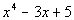, starts out with degree 4, then the next highest degree is 1. It is missing degrees 3 and 2.  So if we were to put it inside a division box we would write it like this: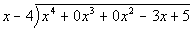.  This will allow you to line up like terms when you go through the problem.  When you set this up using synthetic division write c for the divisor x - c.  Then write the coefficients of the dividend to the right, across the top.  Include any 0's that were inserted in for missing terms.Step 2:  Bring down the leading coefficient to the bottom row.Step 3:  Multiply c by the value just written on the bottom row.

 Place this value right beneath the next coefficient in the dividend:Step 4:  Add the column created in step 3.

 Write the sum in the bottom row:Step 5:  Repeat until done.Step 6: Write out the answer.

 The numbers in the last row make up your coefficients of the quotient as well as the remainder.  The final value on the right is the remainder.  Working right to left, the next number is your constant, the next is the coefficient for x, the next is the coefficient for x squared, etc... The degree of the quotient is one less than the degree of the dividend.  For example, if the degree of the dividend is 4, then the degree of the quotient is 3.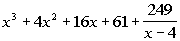Example 1: Divide using synthetic division:.

 Step 1: Set up the synthetic division.

 Long division would look like this: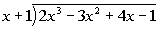Synthetic division would look like this:Step 2:  Bring down the leading coefficient to the bottom row.*Bring down the 2

 Step 3:  Multiply c by the value just written on the bottom row.*(-1)(2) = -2 *Place -2 in next column

 Step 4:  Add the column created in step 3.*-3 + (-2) = -5

 Step 5:  Repeat until done.Step 6: Write out the answer.

 The numbers in the last row make up your coefficients of the quotient as well as the remainder.  The final value on the right is the remainder.  Working right to left, the next number is your constant, the next is the coefficient for x, the next is the coefficient for x squared, etc...Example 2: Divide using synthetic division:Step 1: Set up the synthetic division.

 Long division would look like this: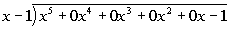Synthetic division would look like this:Step 2:  Bring down the leading coefficient to the bottom row.*Bring down the 1

 Step 3:  Multiply c by the value just written on the bottom row.*(1)(1) =1 *Place 1 in next column

 Step 4:  Add the column created in step 3.*0 + 1 = 1

 Step 5:  Repeat until done.Step 6: Write out the answer.

 The numbers in the last row make up your coefficients of the quotient as well as the remainder.  The final value on the right is the remainder.  Working right to left, the next number is your constant, the next is the coefficient for x, the next is the coefficient for x squared, etc...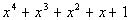Remainder Theorem If the polynomial f(x) is divided by x - c, then  the reminder is f(c).

 This means that we can apply synthetic division and the last number on the right, which is the remainder, will tell us what the functional value of c is.Example 3: Given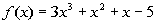, use the Remainder Theorem to find f(-2).

 The steps to the synthetic division are the same as described above.  What is different is what are final answer is going to be.  This time, we are looking for the functional value, so our answer will not be a quotient, but only the reminder.   Using synthetic division to find the remainder we get: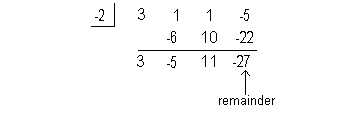Again, our answer this time is not a quotient, but the remainder. Final answer: f(-2) = -27

 Factor Theorem If f(x) is a polynomial AND 1) f(c) = 0, then x - c is a factor of f(x). 2) x - c is a factor of f(x), then f(c) = 0.

 Keep in mind that the division algorithm is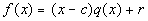dividend = divisor(quotient)+ reminder So if the reminder is zero, you can use this to help you factor a polynomial.  If x - c is a factor, you can rewrite the original polynomial as (x - c) (quotient). You can use synthetic division to help you with this type of problem.  The Remainder Theorem states that f(c) = the remainder.  So if the remainder comes out to be 0 when you apply synthetic division, then x - c is a factor of f(x).Example 4: Use synthetic division to divideby  x - 2.  Use the result to find all zeros of f.

 The steps to the synthetic division are the same as described above.  What is different is what are final answer is going to be.  This time, we are looking for all of the zeros of f.  We will start by dividing using synthetic division and then rewrite f(x) as (x - 2)(quotient).   Using synthetic division to find the quotient we get:Note how the remainder is 0.  This means that (x - 2) is a factor of.

 Rewriting f(x) as (x - 2)(quotient) we get: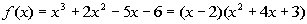We need to finish this problem by setting this equal to zero and solving it: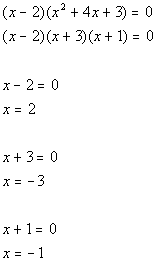*Factor the trinomial   *Set 1st factor = 0   *Set 2nd factor = 0     *Set 3rd factor = 0

 The zeros of this function are x = 2, -3, and -1.Example 5: Solve the equation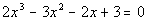given that 3/2 is a zero (or root) of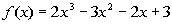.

 The steps to the synthetic division are the same as described above.  What is different is what are final answer is going to be.  This time, we are looking for all of the zeros of f.  We will start by dividing using synthetic division and then rewrite f(x) as (x - 3/2)(quotient).   Using synthetic division to find the quotient we get:Note how the remainder is 0.  This means that (x - 3/2) is a factor of.

 Rewriting f(x) as (x - 3/2)(quotient) we get: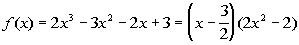We need to finish this problem by setting this equal to zero and solving it: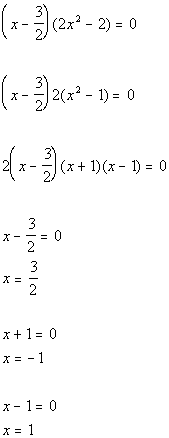*Factor the difference of squares *Note the 1st factor is 2, which is a constant,  which can never = 0 *Set 2nd factor = 0       *Set 3rd factor = 0     *Set 4th factor = 0

 The solution or zeros of this function are x = 3/2, -1, and 1.Practice Problems

 These are practice problems to help bring you to the next level.  It will allow you to check and see if you have an understanding of these types of problems. Math works just like anything else, if you want to get good at it, then you need to practice it.  Even the best athletes and musicians had help along the way and lots of practice, practice, practice, to get good at their sport or instrument.  In fact there is no such thing as too much practice. To get the most out of these, you should work the problem out on your own and then check your answer by clicking on the link for the answer/discussion for that  problem.  At the link you will find the answer as well as any steps that went into finding that answer.Practice Problem 1a: Divide using synthetic division.

 1a.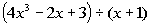(answer/discussion to 1a)Practice Problem 2a: Given the function f(x), use the Remainder Theorem to find f(-1).

 2a.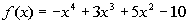(answer/discussion to 2a)Practice Problem 3a: Solve the given equation given that 1/2 is a zero (or root) of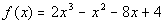.

 3a.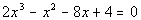(answer/discussion to 3a)Need Extra Help on these Topics?

The following are webpages that can assist you in the topics that were covered on this page:

 http://www.purplemath.com/modules/synthdiv.htm This webpage helps you with synthetic division. http://www.purplemath.com/modules/remaindr.htm This webpage helps you with the Remainder Theorem. http://www.purplemath.com/modules/factrthm.htm This webpage helps you with the Factor Theorem.

Last revised on March 15, 2012 by Kim Seward.
All contents copyright (C) 2002 - 2012, WTAMU and Kim Seward. All rights reserved.